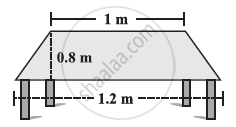# The Shape of the Top Surface of a Table is a Trapezium. Find Its Area If Its Parallel Sides Are 1 M and 1.2 M and Perpendicular Distance Between Them is 0.8 M. - Mathematics

The shape of the top surface of a table is a trapezium. Find its area if its parallel sides are 1 m and 1.2 m and perpendicular distance between them is 0.8 m.#### Solution

Area of trapezium = 1/2 (Sum of parallel sides) × (Distances between parallel sides)

=[1/2 (1+1.2)(0.8)]m^2 = 0.88 m^2

Concept: Area of Trapezium
Is there an error in this question or solution?

#### APPEARS IN

NCERT Class 8 Maths
Chapter 11 Mensuration
Exercise 11.2 | Q 1 | Page 177